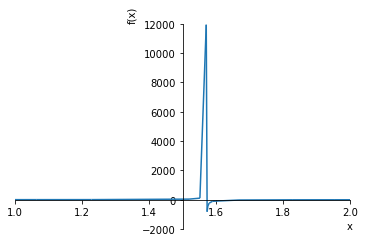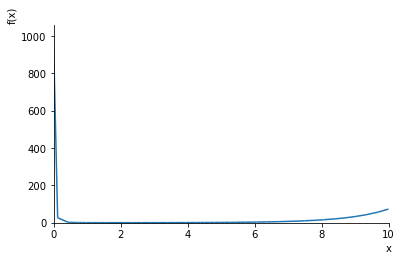## L'Hospital's Rule for Calculating Limits and Indeterminate Forms

L'Hospital's Rule allows us to simplify the evaluation of limits that involve indeterminate forms. An indeterminate form is defined as a limit that does not give enough information to determine the original limit. The most common indeterminate forms that occur in calculus and other areas of mathematics include:

$$\frac{0}{0}, \qquad \frac{\infty}{\infty}, \qquad 0 \times \infty, \qquad 1^\infty, \qquad \infty - \infty, \qquad 0^0, \qquad \infty^0$$

L'Hospital's Rule can be stated as the following:

Suppose $f$ and $g$ are both differentiable functions that approach $0$ (or $\pm \infty$) as $x \rightarrow a$. Then $\frac{f(x)}{g(x)}$ approaches the same limit as $\frac{f^\prime(x)}{g^\prime(x)}$.

The derivatives on the numerator and denominator are both taken separately.

## Examples¶

In :
from sympy import symbols, limit, diff, sin, cos, log, tan, sqrt, init_printing, plot, oo
from mpmath import ln, e, pi

init_printing()
x = symbols('x')
y = symbols('y')


### Example 1: Find the limit of $\lim_{x \rightarrow 1} \frac{x^2 - 1}{x^2 - x}$¶

We see the limit becomes the indeterminate form $\frac{0}{0}$ as $x \rightarrow 1$. Applying L'Hospital's Rule, we differentiate the numerator and denominator separately:

$$\frac{\frac{d}{dx} x^2-1}{\frac{d}{dx} x^2-x}$$

Then carrying the derivation:

$$\frac{\frac{d}{dx} x^2-1}{\frac{d}{dx} x^2-x} = \frac{2x}{2x-1} = \frac{2(1)}{2(1)-1)} = \frac{2}{1} = 2$$

We can confirm our answer using Sympy and the limit() function.

In :
limit((x ** 2 - 1) / (x ** 2 - x), x, 1)

Out:
$$2$$

### Example 2: Find the limit of $\lim_{x \rightarrow 1} \frac{x^9-1}{x^5-1}$¶

As before, start by differentiating both the numerator and denominator:

$$\frac{\frac{d}{dx} x^9-1}{\frac{d}{dx} x^5-1} = \frac{9x^8}{5x^4} = \frac{9(1)^8}{5(1)^4} = \frac{9}{5}$$
In :
limit((x ** 9 - 1) / (x ** 5 - 1), x, 1) # Check our above result

Out:
$$\frac{9}{5}$$

### Example 3: Determine the limit of $\lim_{x \rightarrow (\frac{\pi}{2})^+} \frac{\cos{x}}{1-\sin{x}}$¶

The limit we are evaluating is on the right-hand side, for now we can proceed to calculate the limit as before:

$$\frac{\frac{d}{dx} \cos{x}}{\frac{d}{dx} 1-\sin{x}} = \frac{-\sin{x}}{\cos{x}} = -\tan{x}$$

The limit we are evaluating is therefore equivalent to $\lim_{x \rightarrow \frac{\pi}{2}^+} -\tan{x}$, which approaches $-\infty$ as it is coming from the right-hand side. We can visualize this to confirm by taking advantage of Sympy's plot() function.

In :
plot(cos(x) / (1 - sin(x)), xlim=(1, 2))Out:
<sympy.plotting.plot.Plot at 0x1117779e8>

Although it's a little hard to see as we're doing a very rough plot, we see that the function shoots up to infinity near $\frac{\pi}{2}$ coming from the left-hand side and negative infinity from the right. We can verify our answer again with Sympy. The third argument '+' is added to the limit() function to instruct Sympy to only consider the limit on the right-hand side.

In :
limit(cos(x) / (1 - sin(x)), x, pi / 2, '+')

Out:
$$-\infty$$

### Example 4: Find the limit of $\lim_{x \rightarrow 0} \frac{e^x-1}{x^3}$¶

Proceeding as before by evaluating the derivatives of the numerator and denominator of the limit:

$$\frac{\frac{d}{dx} e^x - 1}{\frac{d}{dx} x^3} = \frac{e^x}{3x^2} = \frac{e^{(0)}}{3(0)^2}$$

Although we arrive at $\frac{1}{0}$ if we were to continue solving, that is not the correct limit. As $x \rightarrow 0$, the function approaches infinity. We can see this behavior by plotting a rough graph of the function.

In :
plot(e ** x / (3 * x ** 2), xlim=(0, 10))Out:
<sympy.plotting.plot.Plot at 0x11212c7f0>

From the graph, we can see that the function shoots up drastically as $x \rightarrow 0$ due to the denominator approaching $0$ quicker than the numerator. We can confirm the actual limit is $\infty$ by checking against SymPy.

In :
limit((e ** x - 1) / x ** 3, x, 0)

Out:
$$\infty$$

## Historical Note¶

Although known as L'Hospital's Rule, the theorem was developed by Johann Bernoulli, the Swiss mathematician, in 1694 and likely introduced to L'Hospital. The publication of L'Hospital's textbook on differential calculus in 1696, the first of its kind, included the theorem and thus L'Hospital's name became attached to the rule.

Indeterminate form. (2017, December 23). In Wikipedia, The Free Encyclopedia. From https://en.wikipedia.org/w/index.php?title=Indeterminate_form&oldid=816695609

Stewart, J. (2007). Essential calculus: Early transcendentals. Belmont, CA: Thomson Higher Education.

Weisstein, Eric W. "L'Hospital's Rule." From MathWorld--A Wolfram Web Resource. http://mathworld.wolfram.com/LHospitalsRule.html